## 近地小行星极短弧定轨的进化算法研究1)

*中国科学院行星科学重点实验室, 紫金山天文台 南京 210034

†月球与行星科学国家重点实验室, 澳门科技大学 澳门 999078

**中国科学院比较行星学卓越创新中心 合肥 230026

## STUDY ON EVOLUTIONARY ALGORITHMS FOR INITIAL ORBIT DETERMINATION OF NEAR-EARTH ASTEROIDS WITH TOO-SHORT-ARC1)

*Key Laboratory of Planetary Sciences$,$ Purple Mountain Observatory$,$ CAS$,$ Nanjing $210034$$, China †State Key Laboratory of Lunar and Planetary Sciences, Macau University of Science and Technology, Macao 999078$$,$ China

**CAS Center for Excellence in Comparative Planetology$,$ CAS$,$ Hefei $230026$$,$ China

 基金资助: 1) 中科院先导B项目.  XDB41000000国家自然科学基金.  11903085国家自然科学基金.  11633009国家自然科学基金.  11273067澳门青年学者计划.  AM201920民用航天预研项目.  D020304民用航天预研项目.  D020302中国科学院创新交叉团队、中国科学院红外探测与成像技术重点实验室基金小行星基金会Abstract

Surveying projects of near-earth asteroids continue to emerge, and obtain massive observation data. However, this pattern makes the obtained arc too short, and the traditional methods have great difficulty in orbit determination and identification with ill-posed problem in itself when the arc is short. Then how to effectively use these short arc is of great significance for discovering, monitoring and evaluating the threat of asteroids. Under the evolutionary algorithms, a calculation framework for too-short-arc is constructed with three-variable $(a,e,M)$ optimization, which keeps the dimensionality low while makes the optimization results no longer rely on observational measurements. The differential evolution algorithm with fewer parameters and simple operation is used to conduct experiments using orbital simulation data of asteroids with different eccentricity, then the optimal solutions and their aggregation regions are analyzed. The large eccentricity orbits will have an impact on the sensitivity of the algorithm search due to its complexity, it is need to reduce the search space to improve the search ability. The results show that the algorithm performs well in small eccentricity problem, and can obtain valid results to provide information for subsequent work. And for large eccentricity problem, while the traditional method fails, the distribution of the algorithm still contains the real solution. For the phenomenon that the optimal solution is not obvious in the global distribution, it can be analyzed by combining the distribution density and fitness value. Further research on the issue of large eccentricity is needed in the future, the influence of different observation positions and observation time on the algorithm should be considered, and calculate by classification.

Keywords： asteroids ; orbit determination ; too-short-arc ; evolutionary algorithm

Li Xinran, Zhao Haibin. STUDY ON EVOLUTIONARY ALGORITHMS FOR INITIAL ORBIT DETERMINATION OF NEAR-EARTH ASTEROIDS WITH TOO-SHORT-ARC1). Chinese Journal of Theoretical and Applied Mechanics[J], 2021, 53(3): 902-911 DOI:10.6052/0459-1879-20-084

## 引言

NASA 在 2005 年提出对至少90%的直径超过 140 m 的近地天体进行编目和特性获取,Pan-STARRS、Catalina、NEOWISE及LSST、NEOCam等大量的近地小行星大视场巡天项目的开展使得巡天能力不断增强, 得到了大量的观测数据. 但同时, 新的观测方式也使得无法对巡天中探测到的每一个目标进行后续的跟踪观测,因此获得的弧长都很短, 通常只有一个晚上的拍摄. 为了提高巡天效率,未来采集的数据将会更为稀疏, 并且轨道参数分布范围很广, 包含众多大偏心率轨道,这些短而稀疏的数据给轨道确定以及识别带来了很大困难. 对于这些过短的观测弧段尤其是大偏心率极短弧段, 利用传统的 Laplace 和 Gauss方法无法进行定轨, 加之短弧定轨本身具有的病态性[12-16],使得定轨难度大幅度增加. 由此, 如何有效利用这些数据对小行星进行极短弧定轨,对巡天项目的充分利用及小行星的探测研究都有着重要意义. 近年来针对这类问题,极短弧定轨的概念被明确提出并成为研究热点.极短弧的具体弧长目前尚无严格的定义,通常无法用经典方法得到合理定轨结果的观测弧段即称为极短弧,以区别于传统意义上的短弧定轨[12-13,17-18].

Ansalone 和Curti针对极短弧定轨问题下天基的模拟资料,应用遗传算法(genetic algorithm, GA), 将观测首末时刻的斜距作为优选变量,使定轨问题转换为一个优化问题, 但采用的参数与通常选法相差较多.王志胜等传算法运用到短弧定轨的问题上来, 研究基于测角资料的卫星短弧定轨. 刘磊等将遗传算法应用于天基的短弧定轨问题, 采用双$\rho$迭代模型,对稀疏数据进行定轨. 李鑫冉和王歆[21-22]对参数调整后将遗传算法应用到了极短弧定轨问题中,在地基的空间目标定轨问题中得到了较好的应用.进化算法在优选问题中可以将生物进化中优胜劣汰的现象应用到对最优解的搜寻中,在探索过程中通过积累经验, 启发式地寻找最终解. 算法已有较为成熟的理论基础,在多个领域都有研究和应用[23-24]. 算法对先验信息的依赖性较小,受数据中的噪声影响也较小, 采用进化算法研究定轨问题已成为新的趋势.除遗传算法外, 进化算法中还包含多种不同进化机制的算法, 算法各有特点和优势,粒子群算法(particle swarm optimization, PSO)、差分进化算法(differentialevolution, DE), 及基于统计学思想的分布估计法(estimation of distributionalgorithm, EDA)等已被应用于解决空间目标的短弧定轨问题,并在近圆轨道下有较好表现.

## 1 进化算法

20 世纪 60 年代进化算法基于模拟自然进化的方法首次被提出, 70 年代出现了相关的理论研究, 直至 21 世纪基本成熟.

PSO算法于 1995 年被 Kennedy 和 Eberhart 提出,源于对鸟类捕食行为的研究, 即鸟类找到食物最简单有效的方法就是搜寻当前距离食物最近的鸟的附近区域.与GA算法不同, 它基于群体智能而不是遗传操作, 利用群体中个体对信息的分享,使整个群体的运动在问题求解空间中产生从无序到有序的演化, 最终获得最优解.

DE方法在 1996 年由 Storn 和 Price提出,是目前最有效的随机参数优选算法之一, 它模拟生物进化,将初始种群中两个个体的向量差作为变异方向, 叠加到第三个个体上,以此产生新个体, 反复迭代使得适应环境的个体被保留下来.不同于遗传算法原本采用二进制编码适用于离散问题的求解,它适用于求解连续变量的优化问题. 算法构造思想借鉴了GA算法和PSO算法,算法保留了GA算法的进化过程,同时以类似于PSO算法中的更新方法替代GA算法中的遗传操作, 因此参数和算子较少,计算复杂性降低.

### 图1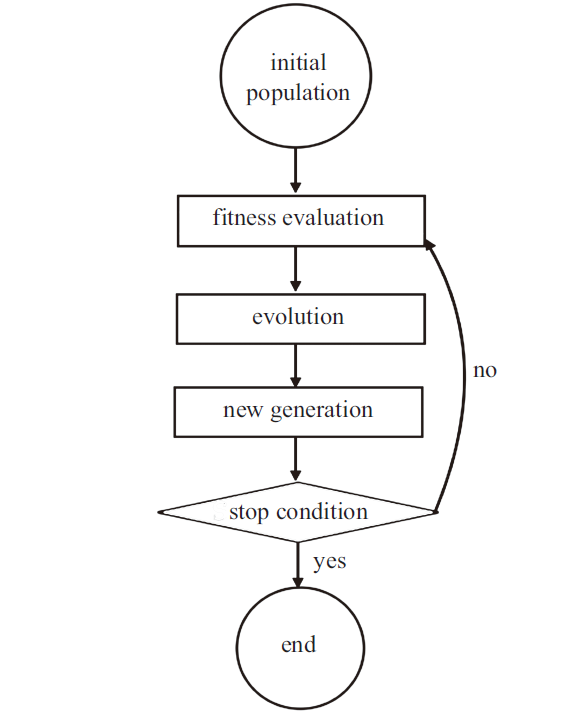Fig. 1   The flowchart of Evolutionary Algorithm

## 2 初轨计算的差分进化算法

DE 算法的主要步骤与GA算法类似, 主要包括变异(mutation)、交叉(crossover)、选择(selection)三种操作,但次序不同. 算法随机生成初始种群${X}=\left\{ {x_{1} ,x_{2} ,...,x_{NP} } \right\}$, 其中$NP$为种群数, $x_{i} =\left\{ {x_{i1} ,x_{i2} ,...,x_{iD} } \right\}$为$D$维向量, $D$为优选变量的维数. DE 算法先进行变异操作, 对每个个体$x_{i}$变异得到个体$V_{i}$,变异方式较GA算法大为简化, 常见的变异方式有以下三种

(1) DE/rand/1

$\begin{eqnarray*} V_{i} =x_{r0} +S(x_{r1} -x_{r2} ) \end{eqnarray*}$

(2) DE/best/1

$\begin{eqnarray*} V_{i} =x_{best} +S(x_{r1} -x_{r2} ) \end{eqnarray*}$

(3) DE/target-to-best/1

$\begin{eqnarray*} V_{i} =x_{i} +S(x_{best} -x_{i} )+S(x_{r1} -x_{r2} ) \end{eqnarray*}$

$\begin{eqnarray*} U_{ij} =\left\{ {\begin{array}{ll} V_{ij} , & \mbox{, } r<CR\ {or}\ j=rand \\ x_{ij} , & \mbox{, } {others} \\ \end{array}} \right. \end{eqnarray*}$

$\begin{eqnarray*} x_{i} (k+1)=\left\{ {\begin{array}{ll} U_{i}, &\mbox{, } F\left( {U_{i} } \right)<F\left( {x_{i} } \right) \\ x_{i} (k), & \mbox{, } {others} \\ \end{array}} \right. \end{eqnarray*}$

## 3 $(a,e,M)$优选法

### 3.3 适值函数

$\left.\begin{array}{l}r_{i}=a\left(1-e \cos E_{i}\right) \\\rho_{i}=\pm \sqrt{r_{i}^{2}-R_{i}^{2}+\left(L_{i} \cdot \boldsymbol{R}_{i}\right)^{2}}-\boldsymbol{L}_{i} \cdot \boldsymbol{R}_{i} \\\boldsymbol{r}_{i}=\rho_{i} \boldsymbol{L}_{i}+\boldsymbol{R}_{i} \\\boldsymbol{R}_{i}=\boldsymbol{R}_{\mathrm{S}}+\boldsymbol{R}_{\mathrm{e}}\end{array}\right\}$

### 图2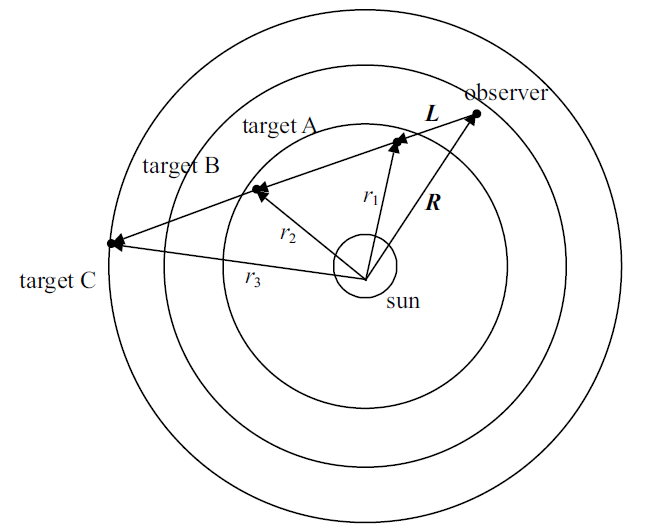Fig. 2   The locations of Earth and asteroid when $R\cdot L<0$

(1) 当$\left| r \right|<\sqrt {R^{2}-(L\cdot R)^{2}}$时,即观测目标距日心的最大距离小于观测路径距离日心的垂直距离, 这显然是不可能的,因此直接剔除此类情况;

(2) 当$\left| r \right|>\left| R \right|$时, 即小行星的轨道高于地球, 处于位置C;

(3) 其他, 此时目标位置有两种可能A或B, 无法根据已有条件确定其具体位置, 需分别计算. 但这一计算非常容易, 不会造成过多负担.

### 图3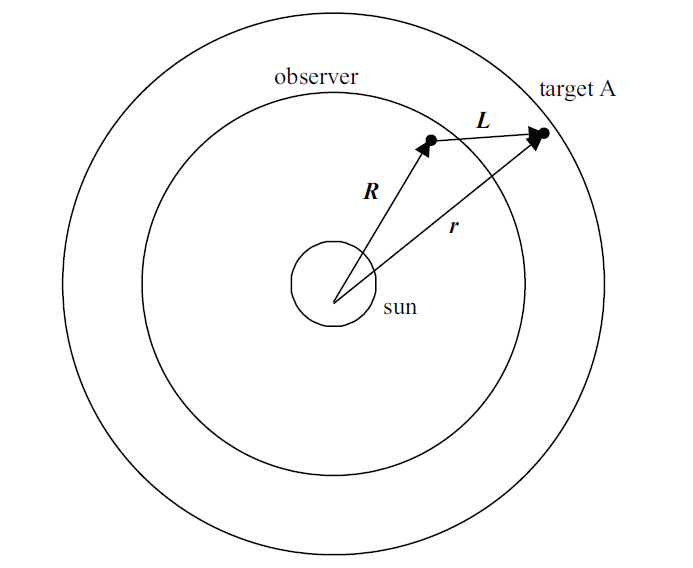Fig. 3   Locations of Earth and asteroid when $R\cdot L>0$

(1) $\left| r \right|<\left| R \right|$时, 目标轨道低于地球, 但考虑到$R$和$L$的夹角, 此情况不可能发生;

(2) 其他, 此时目标只可能位于A, 即轨道在地球之上.

$\begin{eqnarray*} \varDelta_{jk} =f_{k} -f_{j} -\cos^{-1}\left( {\frac{r_{k} \cdot r_{j} }{r_{k} r_{j} }} \right), \\F\left( {x_{i} } \right)=F\left( {(a,e,M)_{i} } \right)=\left( {\frac{2}{N(N-1)}\sum\limits_{j=1}^{N-1} {\sum\limits_{k=j+1}^N {\left( {\Delta _{jk} } \right)^{2}} } } \right)^{\frac{1}{2}} \end{eqnarray*}$

## 4 数值试验

Table 1  The results of orbit determination with measured data and simulated data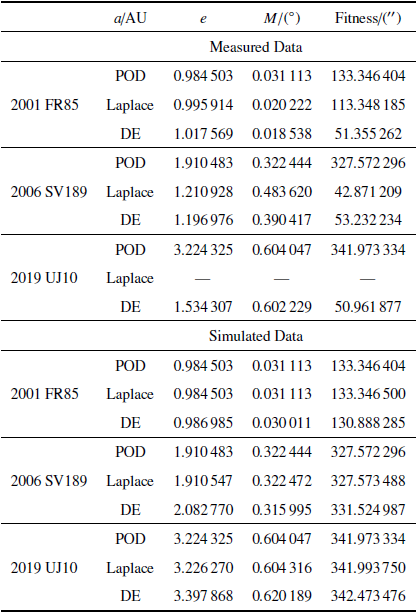Table 2  Orbital elements of asteroids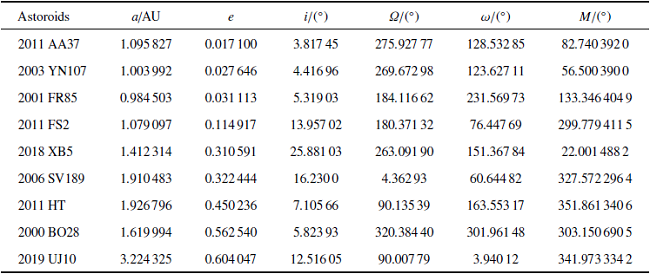### 图4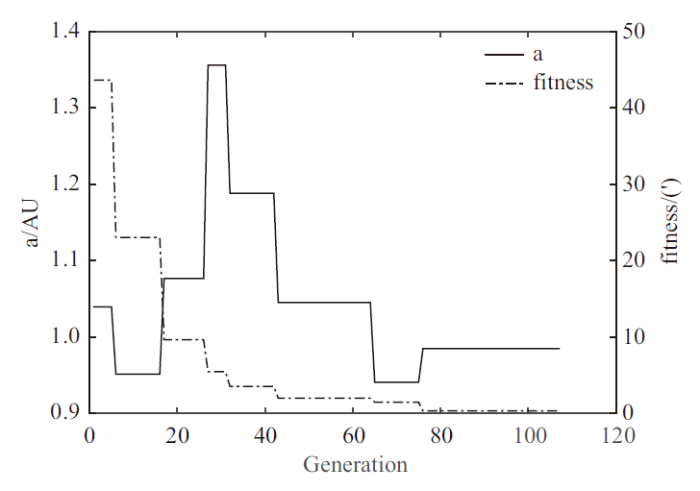Fig. 4   The convergence process of the semi-major and fitness value

Table 3  Orbital Elements of Different Asteroid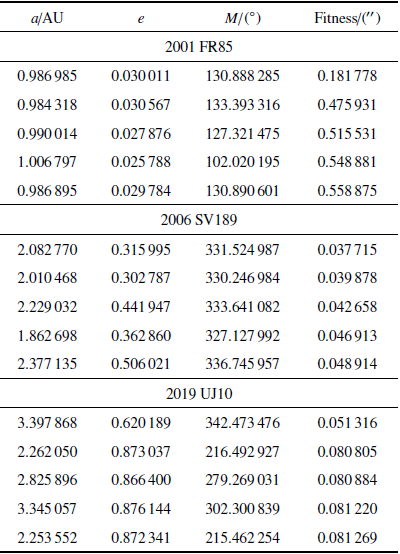### 图5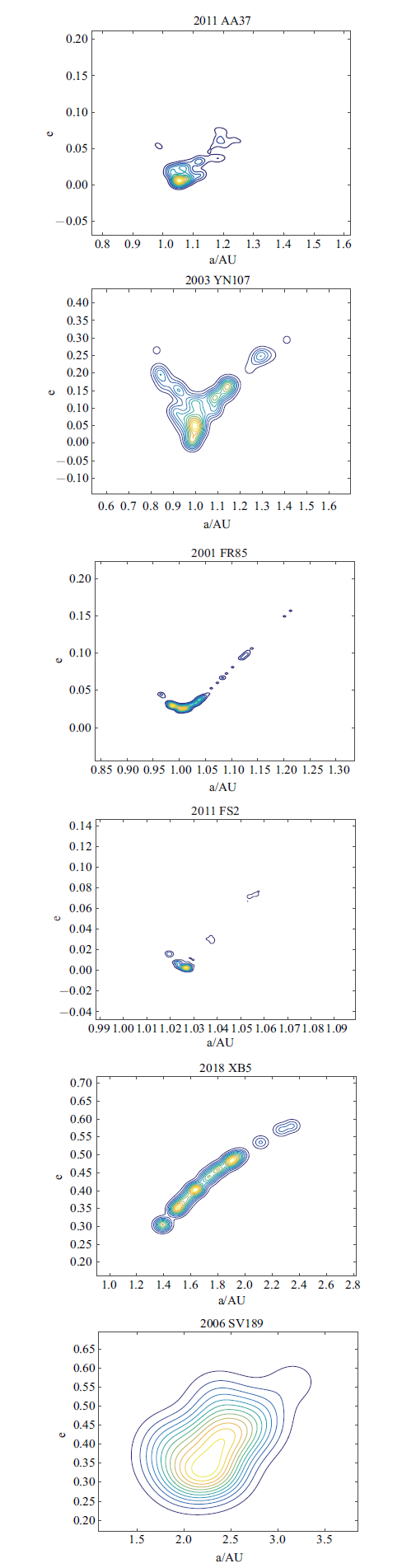Fig. 5   Probability Density

### 图5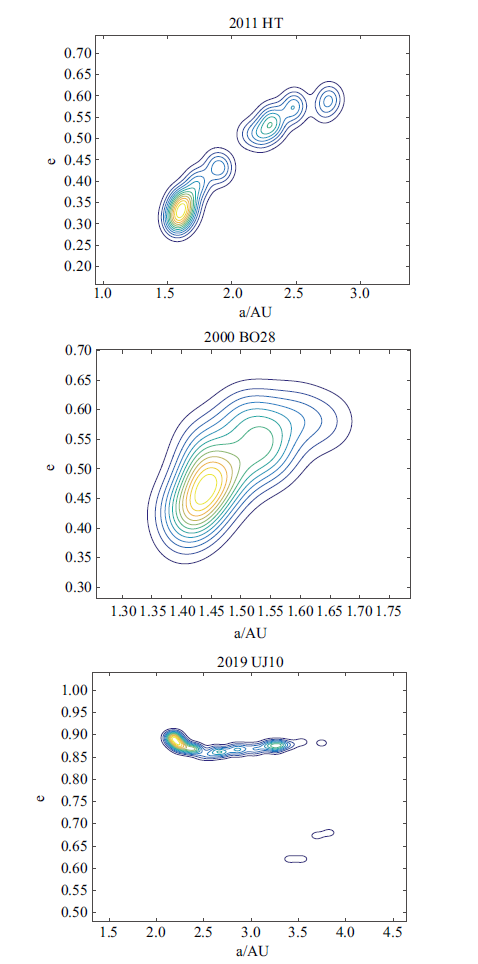Fig. 5   Probability Density (continued)

2011 FS2仅包含半天的观测数据, 而2019 UJ10偏心率较大,其在概率密度分布图上的聚集更加难以显现. 因此将搜索的解空间进行缩小,提高算法的灵敏度, 再次进行试验. 表4中列出了2011 FS2分别在$e\in[0.0,0.3]$和$e\in [0.1,0.2]$内搜寻的结果, 均为适值最小的5个解.可以看到随着搜索区间的缩小, 搜索效率得到提高, 最优解的存在被凸显出来. 将2019UJ10的搜索空间缩小至$e\in [0.6,0.7]$得到了图6的概率密度分布图. 可知,虽然仍有另一个干扰解的存在, 但算法明显搜索到真实解的存在区域,并且在此范围内呈现聚集状. 因此算法在计算大偏心率及过短弧段的轨道时,搜索空间中真实解是存在的, 只是在大范围搜索中不易搜到, 聚集分布不明显.计算时可以通过将区间进行约束划分, 分段计算最优解,来提高算法的灵敏度及搜索能力, 提升聚集程度, 同时, 结果需结合解的分布聚集区域和适值最优的个体考虑.

Table 4  Orbital Elements of 2011 FS2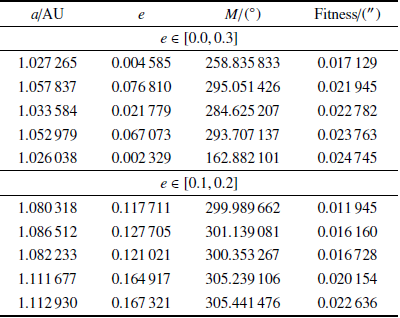### 图6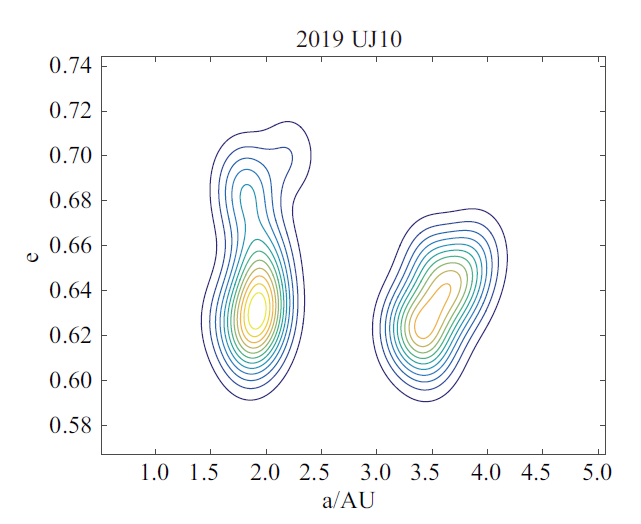Fig. 6   Probability density of $e\in [0.6,0.7]$

Table 5  The results of orbit determination with error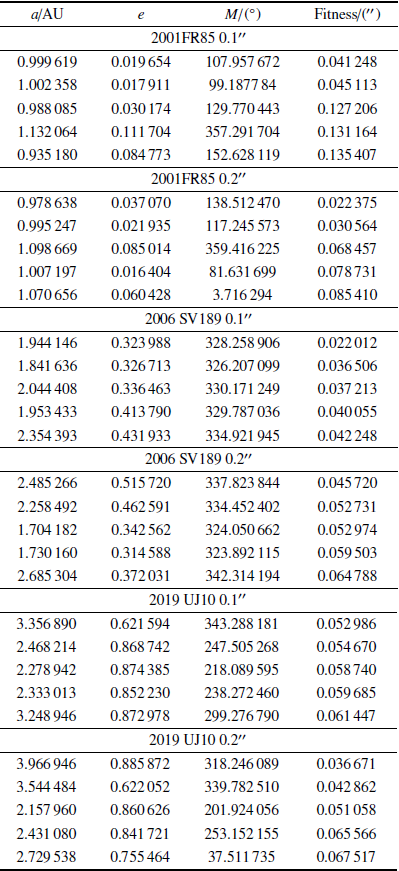### 图7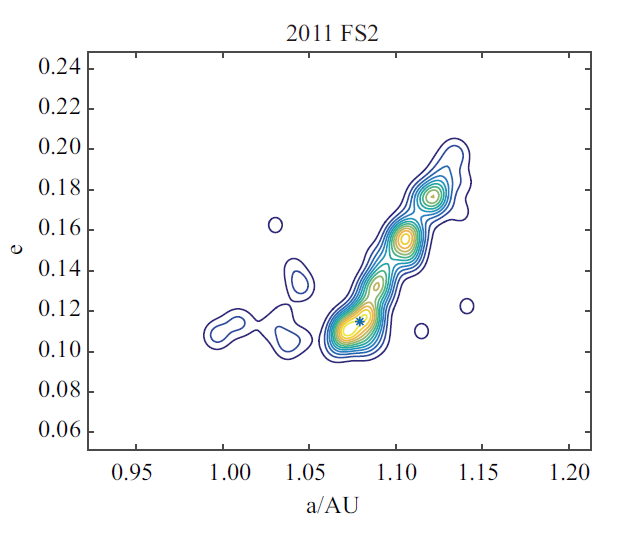Fig. 7   Probability Density of 2011 FS2 with $0.{2}”$ error

## 参考文献 原文顺序 文献年度倒序 文中引用次数倒序 被引期刊影响因子

Schulte P, Alegret L, Arenillas I, et al.

The chicxulub asteroid impact and mass extinction at the cretaceous-paleogene boundary

Science, 2010,327:1214-1218

URL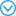The Cretaceous-Paleogene boundary approximately 65.5 million years ago marks one of the three largest mass extinctions in the past 500 million years. The extinction event coincided with a large asteroid impact at Chicxulub, Mexico, and occurred within the time of Deccan flood basalt volcanism in India. Here, we synthesize records of the global stratigraphy across this boundary to assess the proposed causes of the mass extinction. Notably, a single ejecta-rich deposit compositionally linked to the Chicxulub impact is globally distributed at the Cretaceous-Paleogene boundary. The temporal match between the ejecta layer and the onset of the extinctions and the agreement of ecological patterns in the fossil record with modeled environmental perturbations (for example, darkness and cooling) lead us to conclude that the Chicxulub impact triggered the mass extinction.

Proud SR.

Reconstructing the orbit of the Chelyabinsk meteor using satellite observations

Geophysical Research Letters, 2013,40(13):3351-3355

Marcos CDLF, Marcos RDLF.

The Chelyabinsk superbolide: a fragment of asteroid 2011 EO40?

Monthly Notices of the Royal Astronomical Society, 2013,436(1):15-19

Yeomans D, Chodas P.

Possibility of an Earth Impact in 2029 Ruled Out for Asteroid 2004 MN4

NASA's Near Earth Object Program Office, 2029

( Dang Leining, Liu Sen, Bai Zhiyong, et al.

Shi Yilei. Sensitivity research on models of Earth entry and impact effects by asteroids

Chinese Journal of Theoretical and Applied Mechanics, 2021,53(1):278-292 (in Chinese))

Cheng AF, Michel P, Jutzi M, et al.

Asteroid impact & deflection assessment mission: Kinetic impactor

Planetary and Space Science, 2016,121:27-35

Chambers KC, Magnier EA, Metcalfe N, et al.

The Pan-STARRS1 Surveys

astro-ph.IM, 2016, arXiv: 1612.05560

Mikael GA, Robert J.

Debiased orbit and absolute-magnitude distributions for near-Earth objects

Icarus, 2018,312(15):181-207

Mainzer A, Bauer J, Grav T, et al.

The population of tiny near-earth objects by NEOWISE

The Astrophysical Journal, 2014,784:110-116

Grav T, Mainzer AK, Spahr T, et al.

Modeling the performance of the lsst in surveying the near-earth object population

Astronomical Journal, 2016,151(6):172-178

Mainzer A, Grav T, Bauer J, et al.

Survey simulations of a new near-earth asteroid detection system

Astronomical Journal, 2015,149(5):172-189

Milani A, Gronchi GF, Vitturi MD, et al.

Orbit determination with very short arcs. I admissible regions

Celestial Mechanics and Dynamical Astronomy, 2004,90(1):57-85

Milani A, Gronchi GF, Kneević Z, et al.

Orbit determination with very short arcs. II Identifications

Celestial Mechanics and Dynamical Astronomy, 2005,179(2):350-374

( Jia Peizhang, Wu Lianda.

On the optimal accuracy and double solution in the calculation of initial orbits

Chinese Astronomy and Astrophysics, 1999,23(3):384-389 (in Chinese))

( Liu Lin.

Orbit Theory of Spacecraft

Beijing: National Defend Industry Press, 2000

( Wu Lianda, Jia Peizhang.

An analysis of the ill-condition in initial orbit determination

Chinese Astronomy and Astrophysics, 1997,38(3):288-296 (in Chinese))

Tommei G, Milani A, Rossi A.

Orbit determination of space debris: admissible regions

Celestial Mechanics & Dynamical Astronomy, 2007,97(4):289-304

Ansalone L, Curti F.

A genetic algorithm for initial orbit determination from a too short arc optical observation

( Wang Zhisheng, Zhou Jun, Wang Daobo, et al.

A study on intial orbit determination for satellites based on angle measurements

Journal of Projectiles Rockets, Missiles and Guidance, 2002,22(4):190-193 (in Chinese))

URL( Liu L, Xi XN, Rong ZP, et al.

An method and corresponding arithmetic of initial orbit determination based on sparse space-based angle measurements

Journal of Astronautics, 2009,30(3):870-876 (in Chinese))

( Li Xinran, Wang Xin.

Genetic algorithm for initial orbit determination with too short arc

Chinese Astronomy and Astrophysics, 2016,57(1):66-77 (in Chinese))

( Li Xinran, Wang Xin.

Genetic algorithm for initial orbit determination with too short arc (Continued)

Chinese Astronomy and Astrophysics, 2016,57(2):181-187 (in Chinese))

( Xie Chenyue, Yuan Zelong, Wang Jianchun, et al.

Artificial neural network-based subgrid-scale models for large-eddy simulation of turbulence

Chinese Journal of Theoretical and Applied Mechanics, 2021,53(1):1-16 (in Chinese))

( Qin Xia, Liu Shanshan, Shen Yajing, et al.

Rib meshless optimization of stiffened plates resting on elastic foundation based on genetic algorithm

Chinese Journal of Theoretical and Applied Mechanics, 2020,52(1):93-110 (in Chinese))

De Jong KA.

Evolutionary Computation - A Unified Approach

Cambridge: MIT Press, 2006

Kennedy J, Eberhart R.

Particle swarm optimization

// Proceedings of IEEE International Conference on Neural Networks IV. 1995: 1942-1948

Storn R, Price KV.

Differential evolution-A simple and efficient heuristic for global optimization over continuous Spaces

Journal of Global Optimization volume, 1997,11(4):341-359

Rönkkönen J, Kukkonen S, Price KV.

Real-parameter optimization with differential evolution

IEEE Congress on Evolutionary Computation, 2005,1:506-513

Liu J, Lampinen J.

A fuzzy adaptive differential evolution algorithm

Soft Computing, 2005,9(6):448-462

Qin AK, Huang VL, Suganthan PN.

Differential evolution algorithm with strategy adaptation for global numerical optimization

IEEE transactions on Evolutionary Computation, 2009,13(2):398-417

/

 〈〉